## Eigenvalues without determinants

Friday 18 May 2007 at 3:14 pm | In Articles | 9 Comments

Most (all?) undergraduate courses use determinants to introduce eigenvalues and eigenvectors. So the eigenvalues of a matrix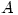(or linear transformation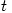) are the solutions of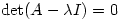. However, Sheldon Axler published a paper in 1994 called Down with Determinants! where he maintains that determinants should not be used so early in linear algebra courses. He gives a very nice proof of the existence of eigenvalues in finite-dimensional vector spaces (over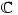) which I would like to reproduce here.

Every linear transformation of a finite-dimensional complex vector space has an eigenvalue.

Here is his proof:

Let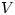be a non-trivial finite-dimensional complex vector space and tÂ a linear transformation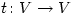. Letbe a fixed non-zero vector inand suppose that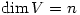. Then thevectors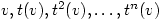are linearly dependent. Hence there exists complex numbers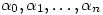not all 0 such that.

and hence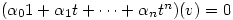.

Now, sinceis algebraically closed, Â the polynomialwill factorise so we get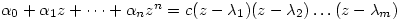.

where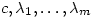are complex numbers with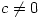. It follows that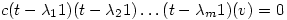which means that, since this is composition of functions, and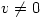, then
either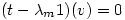soÂ Â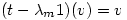Â so thatandis an eigenvector
orsoÂ is an eigenvector
or

oris an eigenvector,
and hencehas an eigenvalue. Â Â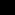Discussion on this approach of not using determinants can be found at NeverEndingBooksÂ and The n-category CafÃ©.

1. My Vector Spaces instructor, when introducing eigenvectors also gave us this little caveat. Most Linear Algebra classes I’ve seen deal with the reals anyway.

By the way, on the line starting “where c=0 and …” you have “c, r_1, …, r_m”. Did you mean lambda instead of r?

Comment by pierre — Friday 18 May 2007 7:38 pm #

2. Whoops! Thanks, yes it should be lambda. I will change it.

The Down with Determinants paper only deals with real vector spaces much later on, considering them as embedded in a complex vector space. He then shows that linear transformations of odd-dimensional real vector spaces have a real eigenvalue.

Comment by steve — Friday 18 May 2007 7:51 pm #

3. I think it’d be nicer if we don’t have to use determinants. For a 4×4 matrix the determinant method can get nasty and my work tends to become messy with a higher chance of errors! It can be ‘tedious’ as well, and then to top it up sometimes it doesn’t factorize nicely!

However like Pierre said, we’ve not really dealt with complex numbers. Everything normally happens in the reals (or sometime Z_p). I think we’re going to be doing Complex Analysis next year.

Comment by beans — Sunday 20 May 2007 4:16 pm #

4. Surely the proof, as stated, isn’t valid. It would imply that any vector v is an eigenvector. Instead what we know is that either v, or (t-λm1)v, or (t-λm-11)(t-λm1)v, etc. is an eigenvector. (Or you could simply point out that you mean “for some v, not equal to the v we chose above” in the last sentence of the proof.)

Nice comment preview BTW!

Comment by sigfpe — Thursday 28 June 2007 11:31 pm #

5. Yes, thanks for that and I will change it. This is the sort of mistake I would make a caustic comment about when a student does it 🙂

Comment by steve — Friday 29 June 2007 10:09 am #

6. Axler has also published an entire book on linear algebra from this point of view. It is called ‘Linear Algebra Done Right’. You may like it. The proofs are quite pretty, but I do not like the book for teaching since it makes it difficult for students to build intuition.

Comment by Scott — Friday 11 January 2008 8:25 pm #

7. Eigenvalues and determinants reveal quite a bit of information about a matrix. In this lab we will learn how to use MATLAB to compute the eigenvalues, eigenvectors, and the determinant of a matrix. We will also learn about diagonalization and how it can be applied to study certain problems in population dynamics.

Comment by plastik — Thursday 7 February 2008 9:35 am #

8. When I first learned about eigenvalues/eigenvectors, and still now when they are mentioned in other classes it is always with determinants. After reading your post I really wish that I had seen this approach since it makes things more obvious (to me at least).

Comment by Nicholas James — Saturday 22 November 2008 7:03 pm #

9. But how do you know that the vectors v, tv, t2v, t3v, …., tnv are all distinct to begin with?

Comment by Angelos Sphyris — Tuesday 13 March 2012 6:21 pm #

Sorry, the comment form is closed at this time.